Next: Results Up: Grammatical Evolution : Solving Previous: Example Individual

# The Problem Space

As proof of concept, we have applied our system to a trigonometric identity problem described by Koza [Koza 92]. The particular function examined was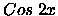, and the desired trigonometric identity was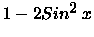. The system was given a set of input and output pairs, and must determine the function that maps one onto the other, with the input values in the range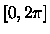. Table  2 contains a tableau which summarizes Koza's experiments.

Table 2: A Koza-style tableau
 Objective : Find a new mathematical expression, in symbolic form that equals a given mathematical expression, for all values of its independent variables. GPTerminal Set: X , the constant 1.0. GPFunction Set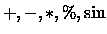Fitness cases The given sample of 20 data points in the interval [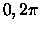] Raw Fitness The sum, taken over the 20 fitness cases, of the error Standardised Fitness Same as raw fitness Hits The number of fitness cases for which the error is less than 0.01 Wrapper None Parameters M = 500, G = 51

Other identities exist for, such as 2 Cos2x - 1. If we were to include Cos as one of the <pre-op> rules, GE would naturally produce simple Cos identities for, which was verified in early experiments which included Cos. These runs consistently found the target expressionand, therefore, we decided to exclude Cos from the terminal operator set. Koza included the constant 1.0 in his terminal operator set, although Genetic Programming has shown an ability to generate the constant 1.0, as has GE with expressions such as x/x. However, we included 1.0 for consistency reasons.
We adopt a similar style to Koza of summarizing information, using a modified version of his tableau in Table  3. Notice how our terminal operands and terminal operators are analogous to GPTerminals and GPfunctions respectively.

Table 3: Grammatical Evolution Tableau
 Objective : Find a new mathematical expression, in symbolic form that equals a given mathematical expression, for all values of its independent variables. Terminal Operands: X , the constant 1.0 Terminal Operators The binary operators +, *, /, and - The unary operator Sin Fitness cases The given sample of 20 data points in the interval [] Raw Fitness The sum, taken over the 20 fitness cases, of the error Standardised Fitness Same as raw fitness Hits The number of fitness cases for which the error is less than 0.01 Wrapper Standard productions to generate C functions Parameters M = 500, G = 51

The production rules for <expr> are as given earlier. As this and subsequent rules are the only ones that require a choice, they are the ones that will be evolved.Next: Results Up: Grammatical Evolution : Solving Previous: Example Individual
root
1998-10-02